NEET  >  Irodov Solutions: Liquids Capillary Effects - 1

# Irodov Solutions: Liquids Capillary Effects - 1 - Notes | Study Physics Class 11 - NEET

 1 Crore+ students have signed up on EduRev. Have you?

Q. 160. Find the capillary pressure
(a) in mercury droplets of diameter d = 1.5 pm;
(b) inside a soap bubble of diameter d = 3.0 mm if the surface tension of the soap water solution is α = 45 mN/m.

Solution. 160.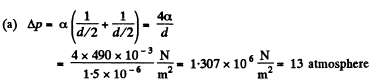(b) The soap bubble has two surfaces

so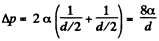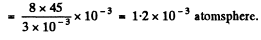Q. 161. In the bottom of a vessel with mercury there is a round hole of diameter d = 70 μm. At what maximum thickness of the mercury layer will the liquid still not flow out through this hole?

Solution. 161.  The pressure just inside the hole will be less than the outside pressure by 4 α/d. This can support a height h of Hg where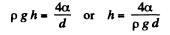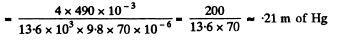Q. 162. A vessel filled with air under pressure P0 contains a soap bubble of diameter d. The air pressure having been reduced isothermally n-fold, the bubble diameter increased η-fold. Find the surface tension of the soap water solution.

Solution. 162. By Boyle's law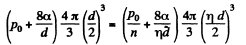or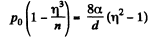Thus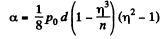Q. 163. Find the pressure in an air bubble of diameter d = 4.0 μm, located in water at a depth h = 5.0 m. The atmospheric pressure has the standard value P0.

Solution. 163. The pressure has terms due to hydrostatic pressure and capillarity and they add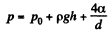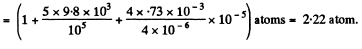Q. 164. The diameter of a gas bubble formed at the bottom of a pond is d = 4.0 μm. When the bubble rises to the surface its diameter increases n = 1.1 times. Find how deep is the pond at that spot. The atmospheric pressure is standard, the gas expansion is assumed to be isothermal.

Solution. 164.  By Boyle's law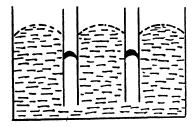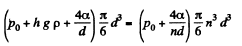or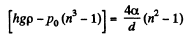or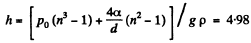meter of water

Q. 165. Find the difference in height of mercury columns in two communicating vertical capillaries whose diameters are d1 = 0.50 mm and d2  = 1.00 mm, if the contact angle θ = 138°.

Solution. 165. Clearly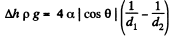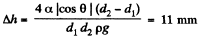Q. 166. A vertical capillary with inside diameter 0.50 mm is submerged into water so that the length of its part protruding over the water surface is equal to h = 25 mm. Find the curvature radius of the meniscus.

Solution. 166. In a capillary with diameter d = 0.5 mm water will rise to a height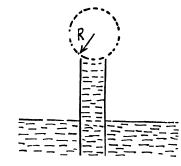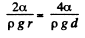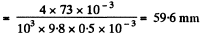Since this is greater than the height (= 25 mm) of the tube, a meniscus of radius R will be formed at the top of the tube, where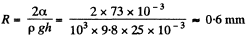Q. 167. A glass capillary of length l = 110 mm and inside diameter d = 20 μm is submerged vertically into water. The upper end of the capillary is sealed. The outside pressure is standard. To what length x has the capillary to be submorged to make the water levels inside and outside the capillary coincide?

Solution. 167. Initially the pressure of air in the cppillary is p0 and it’s length is Z. When submerged under water, the pressure of air in the portion above water must be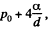since the level of water inside the capillary is the same as the level outside. Thus by Boyle’s law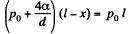or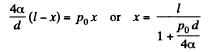Q. 168. When a vertical capillary of length l with the sealed upper end was brought in contact with the surface of a liquid, the level of this liquid rose to the height h. The liquid density is p, the inside diameter of the capillary is d, the contact angle is θ, the atmospheric pressure is P0. Find the surface tension of the liquid.

Solution. 168. We have by Boyle’s law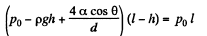or,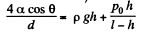Hence,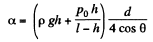Q. 169. A glass rod of diameter d1 =  1.5 mm is inserted symmetrically into a glass capillary with inside diameter d2  = 2.0 mm. Then the whole arrangement is vertically oriented and brought in contact with the surface of water. To what height will the water rise in the capillary?

Solution. 169. Suppose the liquid rises to a height h. Then the total energy of the liquid in the capillary is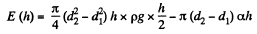Minimising E we get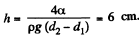Q. 170. Two vertical plates submerged partially in a wetting liquid form a wedge with a very small angle δφ. The edge of this wedge is vertical. The density of the liquid is p, its surface tension is α, the contact angle is θ. Find the height h, to which the liquid rises, as a function of the distance x from the edge.

Solution. 170. Let h be the height of the water level at a distance x from the edge. Then the total eneigy of water in the wedge above the level outside is.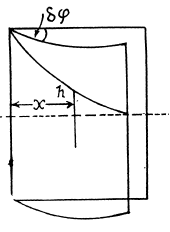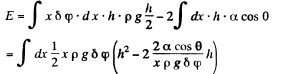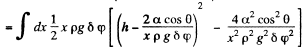This is minimum when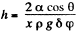Q. 171. A vertical water jet flows out of a round hole. One of the horizontal sections of the jet has the diameter d = 2.0 mm while the other section located l = 20 mm lower has the diameter which is n = 1.5 times less. Find the volume of the water flowing from the hole each second.

Solution. 171. From the equation of continuity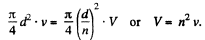We then apply Bernoulli’s theorem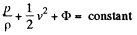The pressure p differs from the atmospheric pressure by capillary effects. At the upper section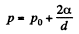neglecting the curvature in the vertical plane. Thus,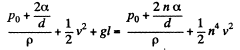or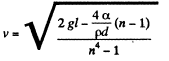Finally, the liquid coming out per second is,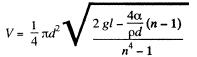Q. 172. A water drop falls in air with a uniform velocity. Find the difference between the curvature radii of the drop's surface at the upper and lower points of the drop separated by the distance h = 2.3 mm.

Solution. 172. The radius of curvature of the drop is R1 at the upper end of the drop and R2 at the lower end. Then the pressure inside the drop is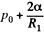at the top end and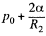at the bottom end. Hence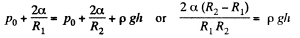To a first approximation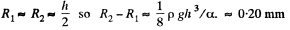if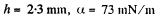The document Irodov Solutions: Liquids Capillary Effects - 1 - Notes | Study Physics Class 11 - NEET is a part of the NEET Course Physics Class 11.
All you need of NEET at this link: NEET

## Physics Class 11

127 videos|464 docs|210 tests

## Physics Class 11

127 videos|464 docs|210 tests

### How to Prepare for NEET

Read our guide to prepare for NEET which is created by Toppers & the best Teachers

Track your progress, build streaks, highlight & save important lessons and more!

,

,

,

,

,

,

,

,

,

,

,

,

,

,

,

,

,

,

,

,

,

;